## on a coordinate plane a line contains the point 2’6 and models a proportional relationship which of the following points are also on the lin

Question

on a coordinate plane a line contains the point 2’6 and models a proportional relationship which of the following points are also on the line

in progress 0
3 weeks 2021-08-25T19:39:00+00:00 1 Answers 0 views 0

1. Given:

On a coordinate plane a line contains the point (2,6) and models a proportional relationship.

To find:

The points which are also on the line.

Solution:

The line models a proportional relationship.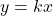…(i)

where, k is constant of proportionality.

Put x=2 and y=6 in the above equation.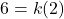Divide both sides by 2.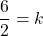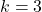Put k=3 in equation (i).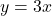We can say that the point whose y-coordinate is 3 times of its x-coordinate, are the point which are lie on the line.

For example: (1,3), (3,3), (-1,-3) etc.

So, the equation of line is. All the points which satisfy this equation represents the points on the line.